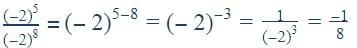# Test: Exponents And Powers- 1

## 10 Questions MCQ Test NCERT Mathematics for CAT Preparation | Test: Exponents And Powers- 1

Description
Attempt Test: Exponents And Powers- 1 | 10 questions in 10 minutes | Mock test for Class 8 preparation | Free important questions MCQ to study NCERT Mathematics for CAT Preparation for Class 8 Exam | Download free PDF with solutions
QUESTION: 1

### (−2)5÷ (−2)8 = ?

Solution:QUESTION: 2

### Write the expression using exponents: 61 × 61 × 61 × 61 × 61

Solution:

Answers is  61^5 because 61 is multiplied 5 times

QUESTION: 3

### Find the value of x, if 32 = 2x.

Solution:

Factorisation of 32 is 2 x 2 x 2 x 2 x 2
32 = 25
X = 5

QUESTION: 4

The repeated factor in an exponential expression is called ____.

Solution:

An expression that represents repeated multiplication of the same factor is called a power. The exponent corresponds to the number of times the base is used as a factor.

QUESTION: 5

A group of students were given an assignment to collect different types of leaves. The group collected32 types of leaves. Represent the number of leaves collected in the form of exponential expression with its base being indivisible.

Solution:

25 = 2 x 2 x 2 x 2 x 2

2 x 2 = 4

4 x 2 = 8

8 x 2 = 16

16 x 2 = 32

QUESTION: 6

Find the value of the expression a2 for a = 10.

Solution:

Multiply 10 twice i.e 10 × 10 because they asked 'a' to the power 2 . so multiply by twice, the solution is 100 .

QUESTION: 7

Evaluate the exponential expression (−b)× (−b)5, for b = 4.

Solution:

(-b)4= (-44) =(-4 × -4) × (-4 × -4)

=16×16 (as -×-=+) =256

(-b)5=1024

so, -1024 × 256 = -262144

QUESTION: 8

When we have to add numbers in standard form, we convert them into numbers with the ________ exponents.

Solution:

For example , 2.38 x 10+ 21.1 x 10= 2.38 x 10+ 2.11 x 10= 4.49 x 104

QUESTION: 9

Simplify and write in exponential form: (−2)−3 × (−2)− 4

Solution:

(-2)-3*(-2)-4 = (-2)(-3)+(-4)  = (-2)(-7)

QUESTION: 10

Evaluate: 82

Solution:

8= 8 x 8 = 64Use Code STAYHOME200 and get INR 200 additional OFF Use Coupon Code